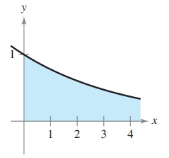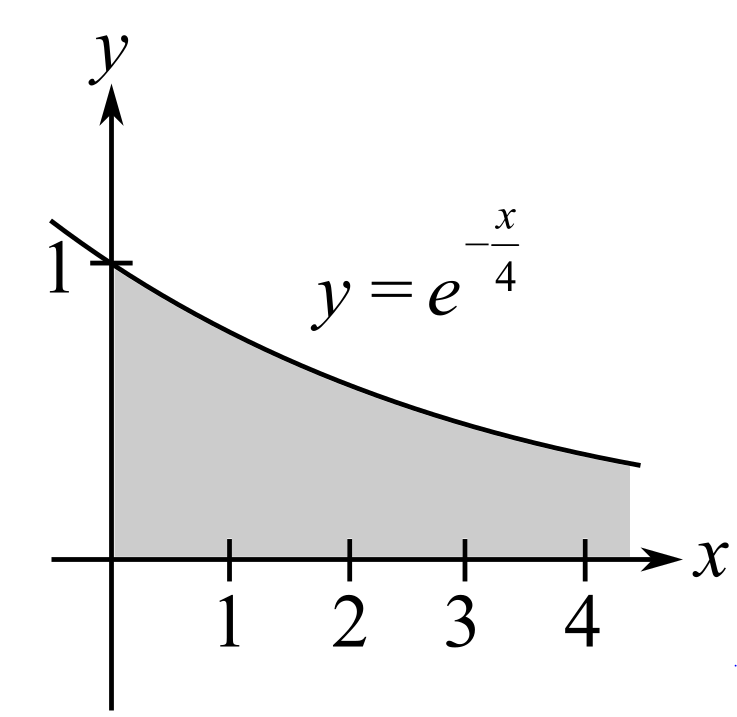Chapter 6, Problem 57RE### Calculus: An Applied Approach (Min...

10th Edition
Ron Larson
ISBN: 9781305860919

#### Solutions

Chapter
Section### Calculus: An Applied Approach (Min...

10th Edition
Ron Larson
ISBN: 9781305860919
Textbook Problem
1 views

# Area of a Region In Exercises 57–60, find the area of the unbounded shaded region. y = e − x / 4To determine

To calculate: The area bounded under the shaded region in the graph of the equation y=ex4.Explanation

Given Information:

The provided equation is,

0ex4dx

And the graph is,

Formula used:

From definition of improper integral.

If on the interval [a,), the function is continuous, then

af(x)dx=limbabf(x)dx

The integral formula:

eaxdx=eaxa+C

Here, a0.

Calculation:

Consider the provided equation:

0ex4dx

And the graph

### Still sussing out bartleby?

Check out a sample textbook solution.

See a sample solution

#### The Solution to Your Study Problems

Bartleby provides explanations to thousands of textbook problems written by our experts, many with advanced degrees!

Get Started

#### In Exercises 23-36, find the domain of the function. 26. g(x)=2x+1x1

Applied Calculus for the Managerial, Life, and Social Sciences: A Brief Approach

#### Which property of real numbers is illustrated in each part of problem 3-6? 4. a) b)

Mathematical Applications for the Management, Life, and Social Sciences

#### What is the partial fraction form of ?

Study Guide for Stewart's Single Variable Calculus: Early Transcendentals, 8th

#### Graph each function. fx=2+lnx

College Algebra (MindTap Course List)